Crises like the COVID-19 pandemic have increased the demand for public health experts who possess advanced analytics skills. After all, data – when properly collected, analyzed and understood – has immense power to inform decision-making. And in areas like public health, informed decision making can save lives. Azhar Nizam has [...]

Because it is near the end of the year, I thought a blog about "Summarizing" data might be in order.

For these examples, I am going to use a simulated data set called Drug_Study, containing some categorical and numerical variables. For those interested readers, the SAS code that I used to generate the data set Drug_Study is shown below. (Please refer to two earlier blogs that describe how to create simulated data sets.)

```*Program to create data set Drug_Study';   proc format; value \$Gender 'M' = 'Male' 'F' = 'Female'; run;   data Drug_Study; call streaminit(13579); length Chol_Group \$ 6; do i = 1 to 1000; do Drug = 'Placebo','A','B'; Subject + 1; if rand('Bernoulli',.5) = 1 then Gender = 'F'; else Gender = 'M';   HR = rand('Normal',80,10) - 10*(Drug = 'A') + 10*(Drug = 'B') - 5*(Gender = 'F'); HR = round(HR);   Cholesterol = rand('Normal',200,20) - 20*(Drug = 'A') -10*(Drug = 'B') - 10*(Gender = 'F'); Cholesterol = round(Cholesterol);   if Cholesterol lt 180 then Chol_Group = 'Low'; else if Cholesterol lt 200 then Chol_Group = 'Normal'; else Chol_Group = 'High';   output; end; end; drop i; format Gender \$Gender.; run;   title "Listing of Drug_Study - first 9 observations"; proc print data=Drug_Study(obs=9); id Subject; var Drug Gender Cholesterol Chol_Group; run;```

The first nine observations from this data set are shown below.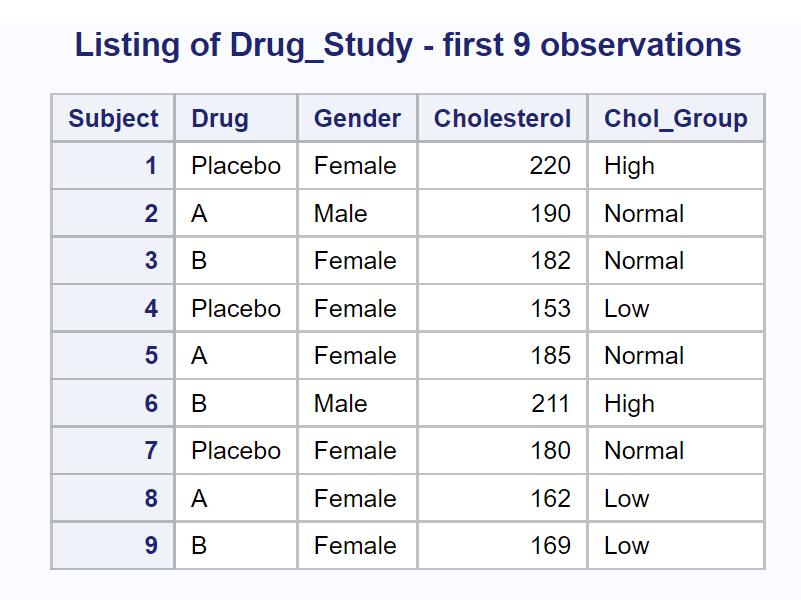Let's start out­ with the most basic summarization—computing statistics for all numeric variables for the entire data set. You can write a program as simple as:

```proc means data=Drug_Study; run;```

However, this program will compute default statistics for every numeric variable in the data set (including Subject). You will probably want to include PROC MEANS options to select what statistics you want and a VAR statement to list the variables that you want to summarize. Here is an example:

```title "Summarizing the Entire Drug_Study Data Set"; proc means data=Drug_Study n nmiss median mean std clm maxdec=3; var HR Cholesterol; run;```

The procedure options selected here are some of my favorites. This is what they represent:

 n The number of nonmissing observations nmiss The number of observations with missing values median The median value (the 50th percentile) mean The mean std The standard deviation clm The 95% confidence limits for the mean. (You are 95% confident that the mean from which this sample was taken is between these two limits.) maxdec The maximum number of places to print following the decimal point

Here is the output: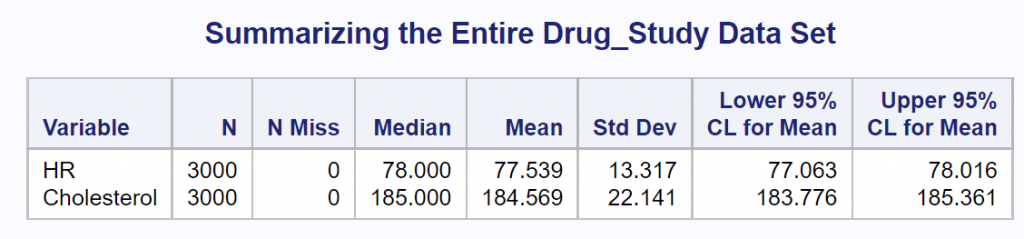You can tell this is simulated (fake) data because there are no missing values. This would be very unusual in a real-life situation.

Most researchers would like to break down the two variables of interest (HR and Cholesterol) by some of the classification variables, such as Gender and Drug. You use a CLASS statement to do this. Let's start out by requesting statistics on HR and Cholesterol, broken down by Drug.

```title "Variables Broken Down by Drug"; proc means data=Drug_Study n nmiss median mean std clm maxdec=3; class Drug; var HR Cholesterol; run;```

Here is the output: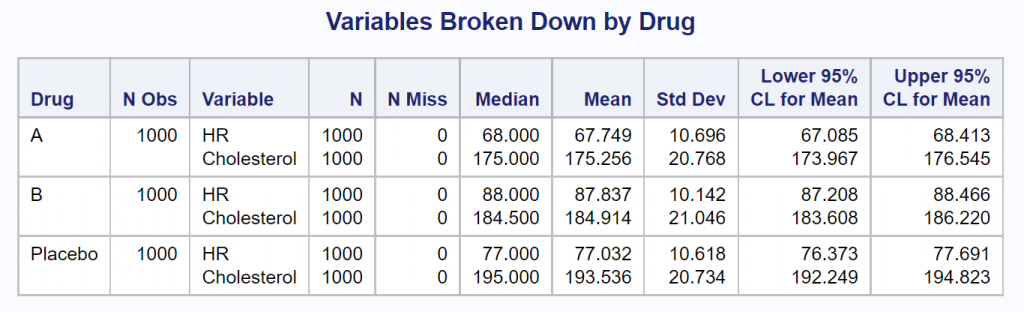You could repeat this program, substituting Gender instead of Drug as the CLASS variable, yielding the following: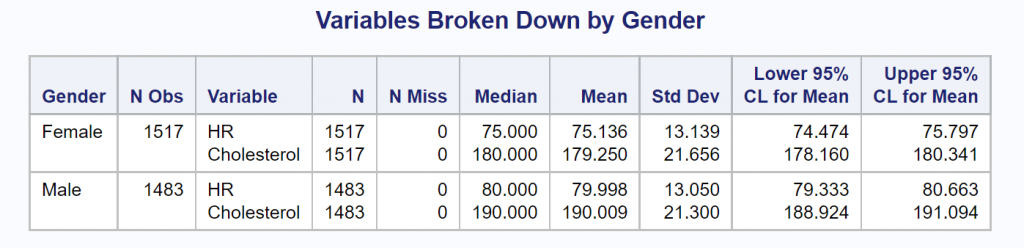Finally, you can include a CLASS statement, listing those two class variables. The program, modified to show statistics broken down by Drug and Gender is shown next, followed by the output.

```title "Variables Broken Down by Drug and Gender"; proc means data=Drug_Study n nmiss median mean std clm maxdec=3; class Drug Gender; var HR Cholesterol; run;```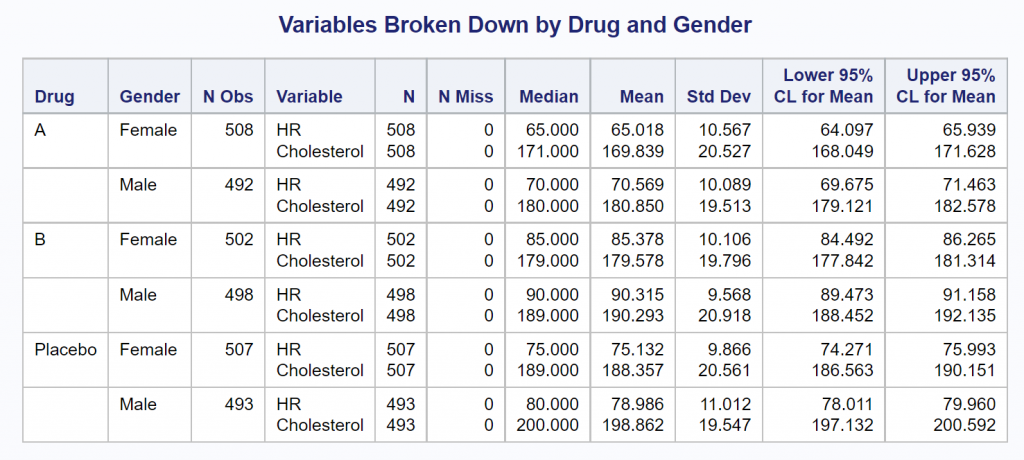It is tedious having to run PROC MEANS four times to produce all of the outputs shown thus far. Instead, you can use the two CLASS variables and add the PROC MEANS option PRINTALLTYPES when you run the program. It looks like this:

```title "Variables Broken Down by Drug and Gender"; footnote "Including the PROC MEANS option PRINTALLTYPES"; proc means data=Drug_Study n nmiss median mean std clm maxdec=3 printalltypes; class Drug Gender; var HR Cholesterol; run;```

In one run, you now see the two variables, HR and Cholesterol, for all combinations of the class variables as shown below.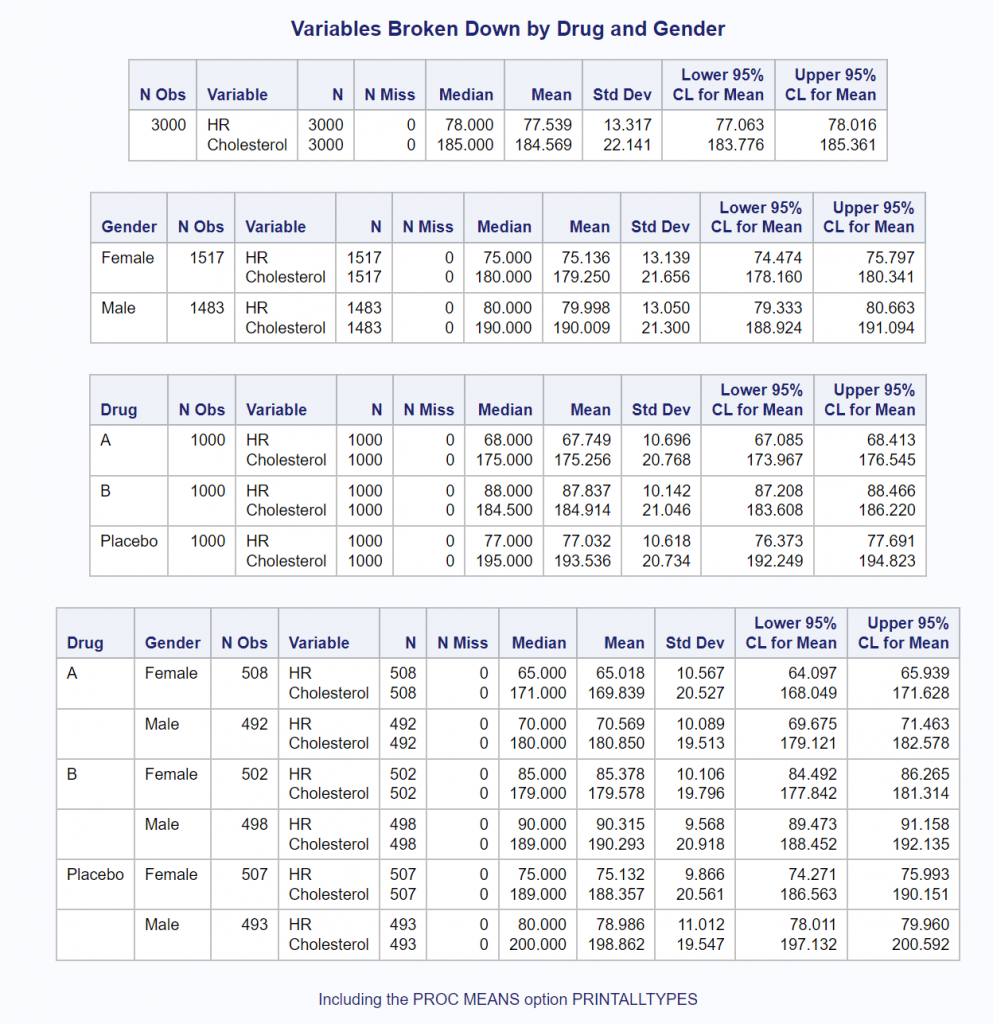All of the programs used in this blog were run using SAS OnDemand for Academics using the SAS Studio editor. You could have also produced all of the outputs using built-in SAS Studio tasks. You can read more about how to do this in my book A Gentle Introduction to Statistics Using SAS Studio in the Cloud.

As always, comments and/or corrections are welcome.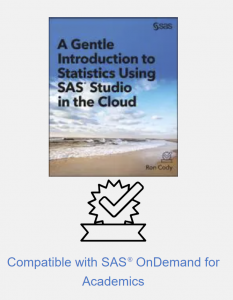Summarizing data was published on SAS Users.

The following is an excerpt from Cautionary Tales in Designed Experiments by David Salsburg. This book is available to download for free from SAS Press. The book aims to explain statistical design of experiments (DOE) to readers with minimal mathematical knowledge and skills. In this excerpt, you will learn about the origin of Thomas Bayes’ Theorem, which is the basis for Bayesian analysis.

The Reverend Thomas Bayes (1702–1761) was a dissenting minister of the Anglican Church, which means he did not subscribe to the full body of doctrine espoused by the Church. We know of Bayes in the 21st century, not because of his doctrinal beliefs, but because of a mathematical discovery, which he thought made no sense whatsoever. To understand Bayes’ Theorem, we need to refer to this question of the meaning of probability.

In the 1930s, the Russian mathematician Andrey Kolomogorov (1904–1987) proved that probability was a measure on a space of “events.” It is a measure, just like area, that can be computed and compared. To prove a theorem about probability, one only needed to draw a rectangle to represent all possible events associated with the problem at hand. Regions of that rectangle represent classes of sub-events.

For instance, in Figure 1, the region labeled “C” covers all the ways in which some event, C, can occur. The probability of C is the area of the region C, divided by the area of the entire rectangle. Anticipating Kolomogorov’s proof, John Venn (1834–1923) had produced such diagrams (now called “Venn diagrams”).

Figure 1 shows a Venn diagram for the following situation: We have a quiet wooded area. The event C is that someone will walk through those woods sometime in the next 48 hours. There are many ways in which this can happen. The person might walk in from different entrances and be any of a large number of people living nearby. For this reason, the event C is not a single point, but a region of the set of all possibilities. The event D is that the Toreador Song from the opera Carmen will resound through the woods. Just as with event C, there are a number of ways in which this could happen. It could be whistled or sung aloud by someone walking through the woods, or it could have originated from outside the woods, perhaps from a car radio on a nearby street. Some of these possible events are associated with someone walking through the woods, and those possible events are in the overlap between the regions C and D. Events associated with the sound of the Toreador Song that originate outside the woods are in the part of region D that does not overlap region C.

The area of region C (which we can write P(C) and read it as “P of C”) is the probability that someone will walk through the woods. The area of region D (which we can write P(D)) is the probability that the Toreador Song will be heard in the woods. The area of the overlap between C and D (which we can write P(C and D) is the probability that someone will walk through the woods and that the Toreador Song will be heard.

If we take the area P(C and D) and divide it by the area P(C), we have the probability that the Toreador Song will be heard when someone walks through the woods. This is called the conditional probability of D, given C. In symbols

P(D|C) = P(C and D)÷ P(C)

Some people claim that if the conditional probability, P(C|D), is high, then we can state “D causes C.” But this would get us into the entangled philosophical problem of the meaning of “cause and effect.”

To Thomas Bayes, conditional probability meant just that—cause and effect. The conditioning event, C, (someone will walk through the woods in the next 48 hours) comes before the second event D, (the Toreador Song is heard). This made sense to Bayes. It created a measure of the probability for D when C came before.

However, Bayes’ mathematical intuition saw the symmetry that lay in the formula for conditional probability:

P(D|C) = P(D and C)÷ P(C) means that

P(D|C)P(C) = P(D and C) (multiply both sides of the equation by P(C)).

But just manipulating the symbols shows that, in addition,

P(D and C) = P(C|D) P(D), or

P(C|D) = P(C and D)÷ P(D).

This made no sense to Bayes. The event C (someone walks through the woods) occurred first. It had already happened or not before event D (the Toreador Song is heard). If D is a consequence of C, you cannot have a probability of C, given D. The event that occurred second cannot “cause” the event that came before it. He put these calculations aside and never sent them to the Royal Society. After his death, friends of Bayes discovered these notes and only then were they sent to be read before the Royal Society of London. Thus did Thomas Bayes, the dissenting minister, become famous—not for his finely reasoned dissents from church doctrine, not for his meticulous calculations of minor problems in astronomy, but for his discovery of a formula that he felt was pure nonsense.

P(C|D) P(D) = P(C and D) = P(D|C) P(C)

For the rest of the 18th century and for much of the 19th century, Bayes’ Theorem was treated with disdain by mathematicians and scientists. They called it “inverse probability.” If it was used at all, it was as a mathematical trick to get around some difficult problem. But since the 1930s, Bayes’ Theorem has proved to be an important element in the statistician’s bag of “tricks.”

Bayes saw his theorem as implying that an event that comes first “causes” an event that comes after with a certain probability, and an event that comes after “causes” an event that came “before” (foolish idea) with another probability. If you think of Bayes’ Theorem as providing a means of improving on prior knowledge using the data available, then it does make sense.

In experimental design, Bayes’ Theorem has proven very useful when the experimenter has some prior knowledge and wants to incorporate that into his or her design. In general, Bayes’ Theorem allows the experimenter to go beyond the experiment with the concept that experiments are a means of continuing to develop scientific knowledge.

To learn more about how probability is used in experimental design, download Cautionary Tales in Designed Experiments now!

Thomas Bayes’ theorem and “inverse probability” was published on SAS Users.One of the first and most important steps in analyzing data, whether for descriptive or inferential statistical tasks, is to check for possible errors in your data. In my book, Cody's Data Cleaning Techniques Using SAS, Third Edition, I describe a macro called %Auto_Outliers. This macro allows you to search for possible data errors in one or more variables with a simple macro call.

### Example Statistics

To demonstrate how useful and necessary it is to check your data before starting your analysis, take a look at the statistics on heart rate from a data set called Patients (in the Clean library) that contains an ID variable (Patno) and another variable representing heart rate (HR). This is one of the data sets I used in my book to demonstrate data cleaning techniques. Here is output from PROC MEANS: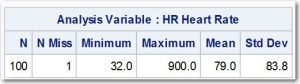The mean of 79 seems a bit high for normal adults, but the standard deviation is clearly too large. As you will see later in the example, there was one person with a heart rate of 90.0 but the value was entered as 900 by mistake (shown as the maximum value in the output). A severe outlier can have a strong effect on the mean but an even stronger effect on the standard deviation. If you recall, one step in computing a standard deviation is to subtract each value from the mean and square that difference. This causes an outlier to have a huge effect on the standard deviation.

### Macro

Let's run the %Auto_Outliers macro on this data set to check for possible outliers (that may or may not be errors).

Here is the call:

```%Auto_Outliers(Dsn=Clean.Patients,
Id=Patno,
Var_List=HR SBP DBP,
Trim=.1,
N_Sd=2.5)```

This macro call is looking for possible errors in three variables (HR, SBP, and DBP); however, we will only look at HR for this example. Setting the value of Trim equal to .1 specifies that you want to remove the top and bottom 10% of the data values before computing the mean and standard deviation. The value of N_Sd (number of standard deviations) specifies that you want to list any heart rate beyond 2.5 trimmed standard deviations from the mean.

### Result

Here is the result: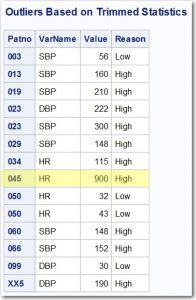After checking every value, it turned out that every value except the one for patient 003 (HR = 56) was a data error. Let's see the mean and standard deviation after these data points are removed.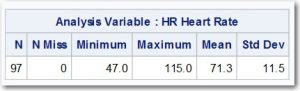Notice the Mean is now 71.3 and the standard deviation is 11.5. You can see why it so important to check your data before performing any analysis.

You can download this macro and all the other macros in my data cleaning book by going to support.sas.com/cody. Scroll down to Cody's Data Cleaning Techniques Using SAS, and click on the link named "Example Code and Data." This will download a file containing all the programs, macros, and data files from the book.  By the way, you can do this with any of my books published by SAS Press, and it is FREE!

Let me know if you have questions in the comments section, and may your data always be clean! To learn more about SAS Press, check out up-and-coming titles, and to receive exclusive discounts make sure to subscribe to the newsletter.

Finding Possible Data Errors Using the %Auto_Outliers Macro was published on SAS Users.Some key components of CASL are the action statements. These statements perform tasks that range from configuring the connection to the server, to summarizing large amounts of data, to processing image files. Each action has its own purpose. However, there is some overlapping functionality between actions. For example, more than one action can summarize numeric variables.
This blog looks at three actions: SIMPLE.SUMMARY, AGGREGATION.AGGREGATE, and DATAPREPROCESS.RUSTATS. Each of these actions generates summary statistics. Though there might be more actions that generate the same statistics, these three are a good place to start as you learn CASL.

### Create a CAS table for these examples

The following step generates a table called mydata, stored in the casuser caslib, that will be used for the examples in this blog.

```cas; libname myuser cas caslib='casuser'; data myuser.mydata; length color \$8; array X{100}; do k=1 to 9000; do i=1 to 50; X{i} = rand('Normal',0, 4000); end; do i=51 to 100; X{i} = rand('Normal', 100000, 1000000); end; if x1 < 0 then color='red'; else if x1 < 3000 then color='blue'; else color='green'; output; end; run;```

### SIMPLE.SUMMARY

The purpose of the Simple Analytics action set is to perform basic analytical functions. One of the actions in the action set is the SUMMARY action, used for generating descriptive statistics like the minimum, maximum, mean, and sum.
This example demonstrates obtaining the sum, mean, and n statistics for five variables (x1–x5) and grouping the results by color. The numeric input variables are specified in the INPUTS parameter. The desired statistics are specified in the SUBSET parameter.

```proc cas; simple.summary / inputs={"x1","x2","x3","x4","x5"}, subset={"sum","mean","n"}, table={caslib="casuser",name="mydata",groupBy={"color"}}, casout={caslib="casuser", name="mydata_summary", replace=true}; run; table.fetch / table={caslib="casuser",name="mydata_summary" }; run; quit;```

The SUMMARY action creates a table that is named mydata_summary. The TABLE.FETCH action is included to show the contents of the table.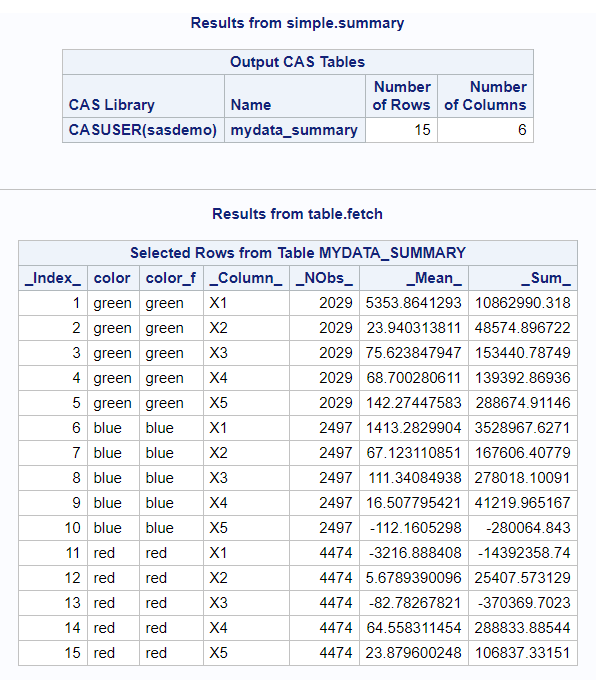The mydata_summary table can be used as input for other actions, its variable names can be changed, or it can be transposed. Now that you have the summary statistics, you can use them however you need to.

### AGGREGATION.AGGREGATE

Many SAS® procedures have been CAS-enabled, which means you can use a CAS table as input. However, specifying a CAS table does not mean all of the processing takes place on the CAS server. Not every statement, option, or statistic is supported on the CAS server for every procedure. You need to be aware of what is not supported so that you do not run into issues if you choose to use a CAS-enabled procedure. In the documentation, refer to the CAS processing section to find the relevant details.
When a procedure is CAS-enabled, it means that, behind the scenes, it is submitting an action. The MEANS and SUMMARY procedure steps submit the AGGREGATION.AGGREGATE action.
With PROC MEANS it is common to use a BY or CLASS statement and ask for multiple statistics for each analysis variable, even different statistics for different variables. Here is an example:

```proc means sum data=myuser.mydata noprint;
by color;
var x1 x2 x3;
output out=test(drop=_type_ _freq_) sum(x1 x3)=x1_sum x3_sum
max(x2)=x2_max std(x3)=x3_std;
run;
```

The AGGREGATE action produces the same statistics and the same structured output table as PROC MEANS.

```proc cas; aggregation.aggregate / table={name="mydata",caslib="casuser",groupby={"color"}} casout={name="mydata_aggregate", caslib='casuser', replace=true} varspecs={{name='x1', summarysubset='sum', columnnames={'x1_sum'}}, {name='x2', agg='max', columnnames={'x2_max'}}, {name='x3', summarysubset={'sum','std'}, columnnames={'x3_sum','x3_std'}}} savegroupbyraw=true, savegroupbyformat=false, raw=true; run; quit;```

The VARSPECS parameter might be confusing. It is where you specify the variables that you want to generate statistics for, which statistics to generate, and what the resulting column should be called. Check the documentation: depending on the desired statistic, you need to use either SUMMARYSUBSET or AGG arguments.

If you are using the GROUPBY action, you most likely want to use the SAVEGROUPBYRAW=TRUE parameter. Otherwise, you must list every GROUPBY variable in the VARSPECS parameter. Also, the SAVEGROUPBYFORMAT=FALSE parameter prevents the output from containing _f versions (formatted versions) of all of the GROUPBY variables.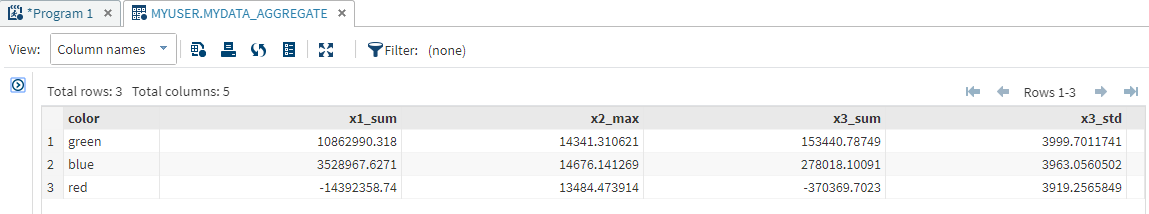### DATAPREPROCESS.RUSTATS

The RUSTATS action, in the Data Preprocess action set, computes univariate statistics, centralized moments, quantiles, and frequency distribution statistics. This action is extremely useful when you need to calculate percentiles. If you ask for percentiles from a procedure, all of the data will be moved to the compute server and processed there, not on the CAS server.
This example has an extra step. Actions require a list of variables, which can be cumbersome when you want to generate summary statistics for more than a handful of variables. Macro variables are a handy way to insert a list of strings, variable names in this case, without having to enter all of the names yourself. The SQL procedure step generates a macro variable containing the names of all of the numeric variables. The macro variable is referenced in the INPUTS parameter.
The RUSTATS action has TABLE and INPUTS parameters like the previous actions. The REQUESTPACKAGES parameter is the parameter that allows for a request for percentiles.
The example also contains a bonus action, TRANSPOSE.TRANSPOSE. The goal is to have a final table, mydata_rustats2, with a structure like PROC MEANS would generate. The tricky part is the COMPUTEDVARSPROGRAM parameter.
The table generated by the RUSTATS action has a column called _Statistic_ that contains the name of the statistic. However, it contains “Percentile” multiple times. A different variable, _Arg1_, contains the value of the percentiles (1, 10, 20, and so on). The values of _Statistic_ and _Arg1_ need to be combined, and that new combined value generates the new variable names in the final table.
The COMPUTEDVARS parameter specifies that the name of the new variable will hold the concatenation of _Statistic_ and _Arg1_. The COMPUTEDVARSPROGRAM parameter tells CAS how to create the values for NEWID. The NEWID value is then used in the ID parameter to make the new variable names—pretty cool!

```proc sql noprint; select quote(strip(name)) into: numvars separated by ',' from dictionary.columns where libname='MYUSER' and memname='MYDATA' and type='num'; quit;   proc cas; dataPreprocess.rustats / table={name="mydata",caslib="casuser"} inputs={&numvars} requestpackages={{percentiles={1, 10, 20, 30, 40, 50, 60, 70, 80, 90, 95 99},scales={"std"}}} casoutstats={name="mydata_rustats",caslib="casuser"} ;   transpose.transpose / table={caslib='casuser', name="mydata_rustats", groupby={"_variable_"}, computedvars={{name="newid",format="\$20."}},computedvarsprogram="newid=strip(_statistic_)||compress(strip(_arg1_),'.-');"} transpose={"_value_"} id={"newid"} casOut={caslib='casuser', name="mydata_rustats2", replace=true}; run; quit;```

Here is a small portion of the final table. Remember, you can use the TABLE.FETCH action to view the table contents as well.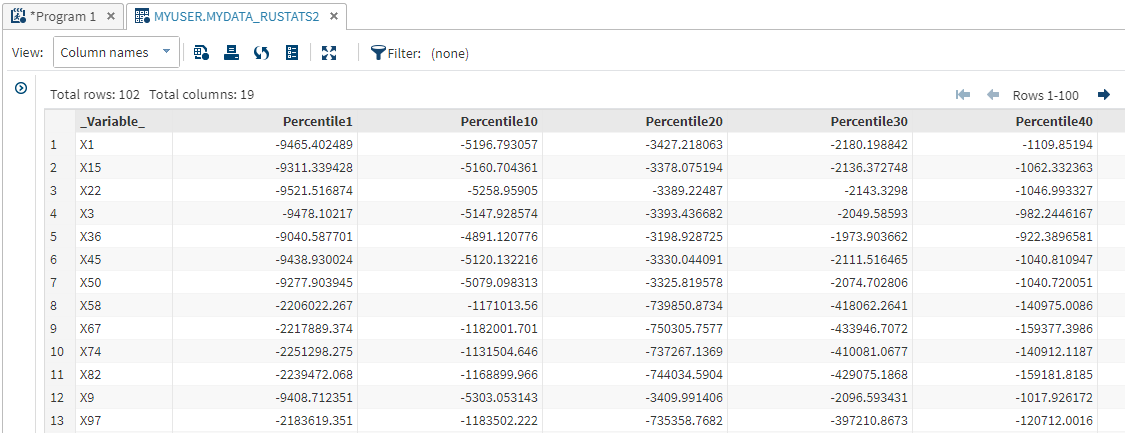### Summary

Summarizing numeric data is an important step in analyzing your data. CASL provides multiple actions that generate summary statistics. This blog provided a quick overview of three of those actions: SIMPLE.SUMMARY, AGGREGATION.AGGREGATE, and DATAPREPROCESS.RUSTATS.
The wonderful part of so many choices is that you can decide which one best fits your needs. Summarizing your data with actions also ensures that all of the processing occurs on the CAS server and that you are taking full advantage of its capabilities.
Be sure to use the DROPTABLE action to delete any tables that you do not want taking up space in memory:

```proc cas; table.droptable / caslib='casuser' name='mydata' quiet=true; table.droptable / caslib='casuser' name='mydata_summary' quiet=true; table.droptable / caslib='casuser' name='mydata_aggregate' quiet=true; table.droptable / caslib='casuser' name='mydata_rustats' quiet=true; table.droptable / caslib='casuser' name='mydata_rustats2' quiet=true; quit; cas casauto terminate;```

Summarization in CASL was published on SAS Users.In the hype and excitement surrounding artificial intelligence and big data, most of us miss out on critical aspects related to collection, processing, handling and analyzing data. It's important for data science practitioners to understand these critical aspects and add a human touch to big data. What are these aspects? [...]Galit Shmueli, National Tsing Hua University’s Distinguished Professor of Service Science, will be visiting the SAS campus this month for an interview for an Analytically Speaking webcast.

Her research interests span a number of interesting topics, most notably her acclaimed research, To Explain or Predict, as well as noteworthy research on statistical strategy, bio-surveillance, online auctions, count data models, quality control and more.

In the Analytically Speaking interview, we’ll focus on her most interesting Explain or Predict work as well as her research on Information Quality and Behavioral Big Data, which was the basis of her plenary talk at the Stu Hunter conference earlier this year. I'll also ask about her books and teaching.

Galit has authored and co-authored many books, two of which — just out this year — include some JMP. First is Data Mining for Business Analytics: Concepts, Techniques, and Applications with JMP Pro, with co-authors, Peter C. Bruce, Nitin R. Patel, and Mia Stephens of JMP. This first edition release coincides with the third edition release of Data Mining for Business Analytics: Concepts, Techniques, and Applications with XLMiner, with the first two co-authors listed above. As Michael Rappa says so well in the foreword of the JMP Pro version of the book, “Learning analytics is ultimately about doing things to and with data to generate insights.  Mastering one's dexterity with powerful statistical tools is a necessary and critical step in the learning process.”

The second book is Information Quality: The Potential of Data and Analytics to Generate Knowledge, which Galit co-authored with Professor Ron S. Kenett, CEO and founder of KPA and research professor at the University of Turin in Italy (you may recognize Ron and KPA colleagues as guest bloggers on the JMP Blog on the topic of QbD). As David Hand notes in his foreword, the book explains that “the same data may be high quality for one purpose and low quality for another, and that the adequacy of an analysis depends on the data and the goal, as well as depending on other less obvious aspects, such as the accessibility, completeness, and confidentiality of the data.”

Both Ron and Galit will be plenary speakers at Discovery Summit Prague in March. You can download a chapter from their book, which discusses information quality support with JMP and features an add-in for Information Quality, both written by Ian Cox of JMP. You can see a short demo of JMP support for information quality during the Analytically Speaking webcast on Nov. 16.

Whether your analysis is seeking to explain some phenomena and/or to make useful predictions, you will want to hear Galit’s thoughtful perspective on the tensions between these two goals, as well as what Galit has to say on other topics up for discussion. Join us! If Nov. 16 doesn’t suit your schedule, you can always view the archived version when convenient.

The post To explain or predict with Galit Shmueli appeared first on JMP Blog.Ronald Snee and Roger Hoerl have written a book called Strategies for Formulations Development. It is intended to help scientists and engineers be successful in creating formulations quickly and efficiently.

The following tip is from this new book, which focuses on providing the essential information needed to successfully conduct formulation studies in the chemical, biotech and pharmaceutical industries:

Although most journal articles present mixture experiments and models that only involve the formulation components, most real applications also involve process variables, such as temperature, pressure, flow rate and so on. How should we modify our experimental and modeling strategies in this case? A key consideration is whether the formulation components and process variables interact. If there is no interaction, then an additive model, fitting the mixture and process effects independently, can be used:

c(x,z) = f(x) + g(z), where 1

f(x) is the mixture model, and g(z) is the process variable model. Independent designs could also be used. However, in our experience, there is typically interaction between mixture and process variables. What should we do in this case? Such interaction is typically modeled by replacing the additive model in Equation 1 with a multiplicative model:

c(x,z) = f(x)*g(z) 2

Note that this multiplicative model is actually non-linear in the parameters. Most authors, including Cornell (2002), therefore suggest multiplying out the individual terms in f(x) and g(z) from Equation 2, creating a linear hybrid model. However, this tends to be a large model, since the number of terms in linearized version of c(x,z) will be the number in f(x) times the number in g(z). In Cornell’s (2002) famous fish patty experiment, there were three mixture variables (7 terms) and three process variables (8 terms), but the linearized c(x,z) had 7*8 = 56 terms, requiring a 56-run hybrid design.

Recent research by Snee et al. (2016) has shown that by considering hybrid models that are non-linear in the parameters, the number of terms required, and therefore the size of designs required, can be significantly reduced, often on the order of 50%. For example, if we fit equation 2 directly as a non-linear model, then the number of terms to estimate is the number in f(x) plus the number in g(z); 7 + 8 = 15 in the fish patty case. Snee et al. (2016) showed using real data that this approach can often provide reasonable models, allowing use of much smaller fractional hybrid designs. We therefore recommended an overall sequential strategy involving initial use of fractional designs and non-linear models, but with the option of moving to linearized models if necessary.

When designing an experiment, a common diagnostic is the statistical power of effects. Bradley Jones has written a number of blog posts on this very topic. In essence, what is the probability that we can detect non-negligible effects given a specified model? Of course, there are a set of assumptions/specifications needed in order to do this, such as effect sizes, error, and significance level of tests. I encourage you to read some of those previous blog posts if you’re unfamiliar with the topic.

If our response is continuous, and we are assuming a linear regression model, we can use results from the Power Analysis outline under Design Evaluation. However, what if our response is based on pass/fail data, where we are planning to do 10 trials at each experimental run? For this response, we can fit a logistic regression model, but we cannot use the results in the Design Evaluation outline. Nevertheless, we’re still interested in the power...

What to do?
We could do a literature review to see about estimating the power, and hope to find something that applies (and do so for each specific case that comes up in the future). But, it is more straight-forward to run a Monte Carlo simulation. To do so, we need to be able to generate responses according to a specified logistic regression model. For each of these generated responses, fit the model and, for each effect, check if the p-value falls below a certain threshold (say 0.05). This has been possible in previous versions of JMP using JSL, but requires a certain level of comfort with scripting and in particular scripting formulas and extracting information from JMP reports. Also, you need to find the time to write the script. In JMP Pro 13, you can now perform Monte Carlo simulations with just a few mouse-clicks.

That sounds awesome
The first time I saw the new one-click simulate, I was ecstatic, thinking of the possible uses with designed experiments. A key element needed to use the one-click simulate feature is a column containing a formula with a random component. If you read my previous blog post on the revamped Simulate Responses in DOE, then you know we already have a way to generate such a formula without having to write it ourselves.

1. Create the Design, and then Make Table with Simulate Responses checked
In this example, we have four factors (A-D), and plan an eight-run experiment. I’ll assume that you’re comfortable using the Custom Designer, but if not, you can read about the Custom Design platform here. This example can essentially be set up the same way as an example in our documentation.

Before you click the Make Table button, you need to make sure that Simulate Responses has been selected from the hotspot at the top of the Custom Design platform.2. Set up the simulation
Once the data table is created, we now have to setup our simulation via the Simulate Response dialog described previously. Under Distribution, we select Binomial, with and set N to 10 (i.e. 10 trials for each row of the design). Here, I’ve chosen a variety of coefficients for A-D, with factor D having a coefficient of 0 (i.e., that factor is inactive). The Simulate Response dialog I will use is:Clicking the Apply button, we get a Y Simulated column simulating the number of successes out of 10 trials, and a column indicating the number of trials (which is used in Fit Model). For modeling purposes, I copied the Y Simulated column into Y.If we look at the formula for Y Simulated, we see that it can generate a response vector based on the model given in the Simulate Responses dialog.3. Fit the Model
Now that we have a formula for simulating responses, we need to set up the modeling for the simulated responses. In this case, we want to collect p-values for the effects from repeated logistic regression analyses on the simulated responses. We first need to do the analysis for a single response. If we launch the Fit Model platform, we can add the number of trials to the response role (Y), and change the Personality to Generalized Linear Model with a Binomial Distribution. My Fit Model launch looks like this:Click the Run button to fit the model. The section of the report that we’re interested in is the Parameter Estimates outline.For the initial simulated response, A, B, and C were found to be active, and D was not (which is correct). Of course, this is just for a single simulation. We could keep simulating a new response vector, and keeping track of these p-values for each effect, or, we could use one-click simulate and let it do this for us.

4. Run the simulation
The column we’re interested in for this blog post is the Prob>ChiSq. We right-click on that column to bring up the menu, and (if you have JMP Pro), at the bottom, above bootstrap, we see an option for Simulate.The dialog that pops up has a choice for Column to Switch Out and a Choice for Column to Switch In. For our simulations, instead of using the response Y, we want to use Y Simulated, as it contains the formula with the Random Binomial. Instead of using Y when we first used Fit Model, we could have instead used Y Simulated, and switch it out with itself. The Number of Samples refers to how many times to simulate a response. Here I’ve left it at 2500.Now we just click OK, and let it run. After a short wait, we’re presented with a data table containing a column for each effect from the Fit Model dialog (as well as a simulation ID, SimID), and 2501 rows – the first is the original fit, and marked as excluded, while each other row corresponds to the results from one of our 2500 simulated responses. The values are the p-values for each effect from Fit Model.The one-click Simulate has also pre-populated the data table with a distribution script, and, because it recognizes the results are p-values, another script called Power Analysis. Running the Power Analysis script provides a distribution of the p-values for each effect, as well as a summary titled Simulated Power with the rejection rate at different levels of alpha. For example, if we look at the effect of factor B, we see that at alpha = 0.05, 2103 times out of 2500 the null hypothesis of no effect was rejected for a rejection rate (empirical power) of about 84%.I typically right-click on one of the Simulated Power tables, and select Make Combined Data Table. This provides a data table that provides the rejection rates for each term at the four different alpha levels. This makes it easier to view the results in Graph Builder, such as the results for alpha = 0.05.Now we can see that we have high power to detect the effects for A and B (recall that they had the largest coefficients), while C and the intercept are around 50%. Since D was inactive in our simulation, the rejection rate is around 0.05, as we would expect. We may be concerned with 50% power assuming that the effect of C is correct. With the ease of being able to perform these simulations, it’s simple to go back to Simulate Reponses and change the number of trials for each row of the design before running another one-click simulation. Likewise, we could create a larger design to see how that affects the power. We could even try modeling using generalized regression with a binomial distribution.

Final thoughts
To me, a key aspect of this new feature is that it allows you to go through different “what if” scenarios with ease. This is especially true if you are in a screening situation, where it’s not unusual to be using model selection techniques when analyzing data. Now you can have empirical power calculations that match the analysis you plan to use, and help alert you to pitfalls that can arise during analysis. While this was possible prior to JMP 13, I typically didn’t find the time to create a custom formula each time I was considering a design. In the short time I’ve been using the one-click simulate, the ease with which I can create the formula and run the simulations has led me to insights I would not have gleaned otherwise, and has become an important tool in my toolbox.

The post Empirical power calculations for designed experiments with 1-click simulate in JMP 13 appeared first on JMP Blog.The Simulate Responses feature throughout various design of experiments (DOE) platforms has always been a useful tool for generating a set of responses according to a specified model. I use it frequently for the simulated responses in Fit Model (or other appropriate platforms), as a way to check that the model is being fit as expected. Prior to JMP 13, Simulate Responses had limitations:

• Simulation was limited to linear regression models with normal errors.
• The ability to simulate responses was tied to the DOE window and the Simulate Responses window. If you closed either window, you would have to make a new data table to simulate responses again.
• If you wanted to run a Monte Carlo simulation study using simulated responses (that is, simulating a large number of responses from the specified model and collecting results), there was no easy way to do so using the simulated responses from the DOE platform.

### Simulate Responses in JMP 13

The look and feel of the Simulate Responses dialog remains the same in JMP 13. But to address the limitations I mentioned above, some new features have been added. That's the focus of the rest of this post.

### Different distributions for the response

There are times when conducting an experiment that the response is not continuous, but instead either pass/fail or count data. In JMP 13, in addition to a linear regression model with normal errors, you now also have the ability to simulate responses that follow a Binomial or Poisson distribution.### Relaunching the Simulate Responses dialog

As discussed above, because Simulate Responses was tied to the DOE platform, there was no easy way to relaunch the Simulate Responses dialog once either was closed. In JMP 13, Simulate Responses is tied to the data table. A table script, called DOE Simulate, relaunches the simulation dialog.### Automatic formula creation

In my view, the most powerful new aspect of the revamped Simulate Responses can be easy to miss. I’ll demonstrate with a simple example, a 12-run Custom Design with four continuous factors (X1-X4). When you select Simulate Responses from the hotspot in a DOE platform and you create a data table, you now end up with two columns for each response that have the same values initially. The second one, Y Simulated (where Y is the name of the response), gets updated each time the Apply button is clicked in the dialog. Why the need for two columns?If you right-click on the column name, you see that Y Simulated is actually a Formula Column. The column Y has no formula – it is simply filled in during the data table creation -- the idea being that you fill your own response values there when collecting data.If you examine the formula, you see the responses are generated based on the model specified in the Simulate Responses dialog:This means that you can also simulate responses by clicking the Apply button within the formula editor. Note that this formula is automatically created by the Simulate Responses dialog. Suppose you change the coefficients and distribution, like this:When you click the Apply button, your Y Simulated column now has count data.The script updates the underlying formula to reflect the new model:### Simulation studies

Why am I so excited about this aspect of Simulate Responses? Simulation studies.
For users comfortable using JSL, the automatic formula only requires the use of Eval Formula to simulate a new response, and then gathering the desired information via scripting. What’s more, if you have JMP Pro 13, this formula allows you to perform one-click Monte Carlo simulations (akin to the one-click bootstrap).

Stay tuned for a blog post by Ryan Parker explaining how to use this new Simulate feature. In a future blog post, I’ll show just how easy it is to perform empirical power calculations using the new Simulate Responses with one-click Simulate.

The post Simulate Responses is revamped to be more useful in JMP 13 appeared first on JMP Blog.